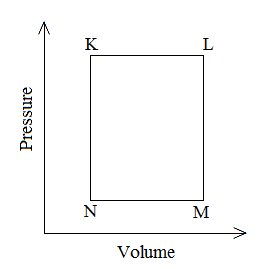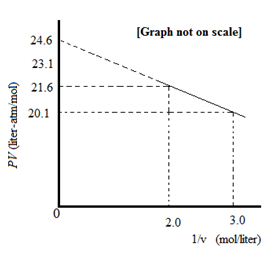×#### Thank you for registering.

One of our academic counsellors will contact you within 1 working day.

Click to Chat

1800-1023-196

+91-120-4616500

CART 0

• 0

MY CART (5)

Use Coupon: CART20 and get 20% off on all online Study Material

ITEM
DETAILS
MRP
DISCOUNT
FINAL PRICE
Total Price: Rs.

There are no items in this cart.
Continue Shopping• Complete JEE Main/Advanced Course and Test Series
• OFFERED PRICE: Rs. 15,900
• View Details

```Question: 1A fixed mass ‘m’ of a gas is subjected to transformation of states from K to L to M to N and back to K as shown in the figureThe pair of isochoric processes among the transformation of states is (IIT JEE-2013)

1) K to L and L to M

2) L to M and N to K

3) L to M and M to N

4) M to N and N to K

Solution: Plot for the processes N to K and L to M are a straight line. This indicates that the value of V remains same at all the points during these processes. These processes take place at constant volume, so these are isochoric processes.

Hence, the correct option is b.Question: 2 For one mole of a van der Waals gas when b = 0 and T= 300 K, the PVvs. 1/V plot is shown below. The value of the van der Waals constant ‘a’ (atm.litre2mol–2) is  (IIT JEE 2012)1) 1.0

2) 4.5

3) 1.5

4) 3.0

Solution:

Van der waals equation for 1 mole of real gas:

(P+a/v2)(v-b) =RT

Given that, b=0

So, the equation becomes;

(P+a/v2)v =RT

or

pv + a/v = RT

or

pv = -a×1/v +RT

Comparing with equation of straight line: y = mx +c, it can be concluded that

pv vs 1/v graph would be a straight line with a negative slope equal to a.

Thus, slope of the plot = a = (y2-y1)/(x2-x1) = (21.6-20.1)/(3-2) =1.5

Hence, the correct option is C.

Question:3 To an evacuated vessel with movable piston under external pressure of 1 atm, 0.1 mol of He and 1.0 mol of an unknown compound (vapour pressure 0.68 atm.at 0oC) are introduced. Considering the ideal gas behaviour, the total volume (in litre) of the gases at 0oC is close to  (IIT-JEE 2011)

Solution

The unknown compound would not follow the ideal gas equation and thus the problem can be solved by applying the ideal gas equation to the He gas only.

Using ideal gas equation:

V = (nRT )/P

V = (0.1×0.0821 × 273)/(0.32)

V = 7 L
```### Course Features

• 728 Video Lectures
• Revision Notes
• Previous Year Papers
• Mind Map
• Study Planner
• NCERT Solutions
• Discussion Forum
• Test paper with Video Solution